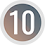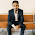# Compound Interest## Compound Interest

Interest is the additional sum of money to be paid for the use of money from a bank or money lender for certain period of time. Similarly, if we keep money in a bank or lend to someone else, we will be paid interest.

Interest is calculated on the basis of the initial sum of money, period of time and the rate of interest. When the interest calculated for certain sum of money is same for every year, it is called simple interest.

But, when the interest of first year is added to the principal to calculate the interest of second year, then it is called compound interest.

### Compound Interest Formula

Compound amount and interest are given by the formula,
where,  CA ---------------> Compound Amount
CI  ---------------> Compound Interest
P  ---------------> Principal (initial sum of money)
T  ---------------> Time (in year)
R  ---------------> Rate of interest (in % per year)

Some other formula,
1.    When the interest is compounded annually with different rates R1 for 1st year, R2 for 2nd year and R3 for 3rd year then,

2.    When the time is given by ‘T’ years and ‘m’ months then,

3.    When interest is compounded half-yearly, then

### Workout Examples

Example 1: Calculate the compound interest and amount when the principal (P) = Rs. 10000, time (T) = 2 years at the rate of interest (R) = 12%.

Solution: Here,
P = Rs. 10000
T = 2 years
R = 12%
We have,

10000 [(1.12)2 – 1]
= 10000 × 0.2544
= 2544

CA = P + CI
= Rs. 10000 + 2544
= Rs. 12544

Example 2: At what rate percent per annum compound interest will Rs. 10000 amounts to Rs. 12544 in 2 years?

Solution: Here,
P = Rs. 10000
CA = Rs. 12544
T = 2 years
We have,
or,        R = 12%

Example 3: In how many years will Rs. 8000 amount to Rs. 13824 at 20% per annum interest compounded yearly?

Solution: Here,
P = Rs. 8000
CA = Rs. 13824
T = ?
We have,
or,        1.728 = (1.2)T
or,        (1.2)3 = (1.2)T
∴          T = 3 years
The required time is 3 years.

Example 4: Find the difference between compound interest compounded semi-annually and the interest compounded annually on Rs. 8000 at 10% per annum in 1.5 years.

Solution: Here,
P = Rs. 8000
R = 10%
T = 1.5 years (1 year 6 months)
Now,
= 8000 (1.053 – 1)
= 8000 × 0.157625
= Rs. 1261

= 8000 (1.1×1.05 – 1)
= 8000 × 0.155
= Rs. 1240

Difference between CI1 and CI2 = Rs. 1261 – 1240 = Rs. 21

Example 5: The compound amount of a sum of money in 3 years is Rs. 13310 and in 4 years is Rs 14641. Find the compound rate of interest per annum and the sum.

Solution: Here,
CA in 3 years = Rs. 13310

or,        R = 10%

Again, putting R = 10 in equation (i), we get,
or,        P (1.1)3 = 13310
or,        P = 13310/1.331
or,        P = Rs. 10000

The required sum is Rs. 10000 and rate of interest is 10% per annum.

You can comment your questions or problems regarding the compound interest here.

1.Prativa deposit rs 150000 in a bank at the rate of 10 percent p.a. pramila deposit 150000 in bank at the rate of 12 percent p.a. if prativa received semi compound interest, which interest is more and by how much in 3 years

1.Here is the solution to your problems,
[image src="https://1.bp.blogspot.com/-hxSpEMfLmOY/YVxpD0wlkxI/AAAAAAAAKI8/6pDUmPlx6i0qzNYfTQai3TZMQeq7oV2DQCLcBGAsYHQ/s16000/comment%2Bsolution%2B1.png "/]

2.आपने मूलधन से ब्याज कैसे निकालते है की जानकारी बहुत अच्छे से दी है . बहुत ही अच्छे उदारण से यह समझ आ गया .

3.The difference of compound interest and the simple interest at the end of 2
years with 20% yearly rate of interest is Rs. 560. What is the simple interest ?

1.Solution:
Here,
T = 2 years
R = 20%

CI – SI = Rs. 560
i.e. P[(1+R/100)^T – 1] – PTR/100 = Rs. 560
or, P[(1 + 20/100)^2 – 1 – 2×20/100] = 560
or, P [1.44 – 1 – 0.4] = 560
or, P × 0.04 = 560
or, P = 560/0.04
or, P = Rs. 14000

Now,
SI = PTR/100
= 14000×2×20/100
= Rs. 5600

4.The compound interest on a sum of money for 2 years and 3 years are Rs.5250 and Rs.8275 respectively. find the principle and rate of interest.